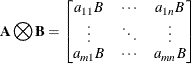The SSM Procedure

Predefined Structural Models

Subsections:

A set of predefined models is available in the SSM procedure for models called structural models in the time series literature. These predefined models can be used to model trend, seasonal, and cyclical patterns in the univariate and multivariate time series. For the most part, the multivariate models are straightforward generalizations of the corresponding univariate models—for example, the multivariate random walk trend described later in this section generalizes the univariate random walk trend that is described in the section Random Walk Trend. All of these models, with the exception of the continuous-time cycle model, are applicable only to the regular data type. The continuous-time cycle model is applicable to all the data types; however, it is available for the univariate case only.

To specify these models, you must first use the STATE statement with the correct TYPE= option. When you specify the TYPE=option, you do not need to specify other options of the STATE statement (for example, the T option, the COV1 option, and the A1 option). However, you must specify the COV option, which describes the covariance of the disturbance term that drives the state equation. Throughout this section, the symmetric matrix specified by using the COV option is denoted by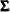. For TYPE= LL, an additional matrix, specified by using the SLOPECOV suboption, also plays a role; it is denoted by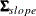. Subsequently you must specify one or more COMPONENT statements to define the (univariate) components that are based on this state subsection for their inclusion in the MODEL statement. These univariate components exhibit interesting behavior based on the structure of(and, whenever applicable)—for example, imposing rank restrictions onin the multivariate random walk results in these univariate trends moving together. For additional information about these models, see Harvey (1989).

The following example summarizes the steps needed to define a multivariate structural model by using a sequence of STATE and COMPONENT statements. For a full example, see Example 27.1. Suppose that a three-dimensional time series is being studied with response variables y1, y2, and y3. Suppose you want to specify the trivariate structural model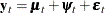where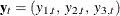denotes the response series, and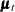,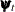, and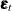denote the trivariate components, trend, cycle, and white noise, respectively. The three components of, the observation noise in the model, are not assumed to be independent. Therefore, you cannot specify them by using three IRREGULAR statements; you must include them in the state specification. The following (incomplete) statements show how to specify this model:

     state whiteNoise(3)  type=wn ...;
component wn1 =  whiteNoise;
component wn2 =  whiteNoise;
component wn3 =  whiteNoise;

state randomWalk(3)  type=rw ...;
component rw1 =  randomWalk;
component rw2 =  randomWalk;
component rw3 =  randomWalk;

state cycleState(3)  type=cycle ...;
component c1 = cycleState;
component c2 = cycleState;
component c3 = cycleState;

model y1 = rw1 c1 wn1;
model y2 = rw2 c2 wn2;
model y3 = rw3 c3 wn3;


The first STATE statement defines whiteNoise, a state subsection that is needed for defining a three-dimensional white noise component. In turn, whiteNoise is used to define the three univariate white noise components: wn1, wn2, and wn3. The components wn1, wn2, and wn3 are correlated—their correlation structure is controlled by the covariance specification of whiteNoise. The second set of STATE and COMPONENT statements result in three correlated random walk trend components: rw1, rw2, and rw3. Finally, the last set of STATE and COMPONENT statements result in three correlated cycle components: c1, c2, and c3. In the end, the desired multivariate model is defined by including these (univariate) components in the appropriate MODEL statements.

In the preceding example, it is important to note the relationship between the nominal dimension (denoted by dim throughout this section) that is specified in the STATE statement and the actual dimension of the resulting state subsection. Note that the three state subsections, whiteNoise, randomWalk, and cycleState, are defined by using the same dim specification: 3. However, the actual dimensions of these state subsections depend on their type; they do not need to equal this specified dimension. Here, whiteNoise and randomWalk do have the same size, 3, as the specified dim. However, the size of cycleState, which is of TYPE=CYCLE, is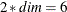. Another important point to note: no matter what the underlying size of the state subsection, the desired univariate components were obtained by using an identical specification scheme in the COMPONENT statement. This happens because the component specification style that is based on the element operator—[]—in the COMPONENT statement behaves differently when the TYPE= option is used to define the state subsection (see the section Multivariate Season for an illustration).

The system matrices for all these models are time-invariant, with the exception of the continuous-time cycle model. In this section,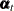denotes the subsection of the overall model state, and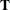,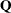, and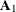denote the corresponding blocks of the larger system matrices.

For the multivariate cycle system matrices described in the section Multivariate Cycle, the Kronecker product notation is useful: if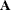is anmatrix and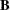is a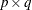matrix, then the Kronecker product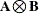is an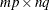block matrix: